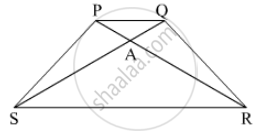Advertisement Remove all ads

# In Trapezium Pqrs, Side Pq || Side Sr, Ar = 5ap, as = 5aq Then Prove That, Sr = 5pq - Geometry

Advertisement Remove all ads
Advertisement Remove all ads
Advertisement Remove all ads

In trapezium PQRS, side PQ || side SR, AR = 5AP, AS = 5AQ then prove that, SR = 5PQAdvertisement Remove all ads

#### Solution

Given:
side PQ || side SR
AR = 5AP,
AS = 5AQ
To prove: SR = 5PQ
Proof: In ∆APQ and ∆ARS
∠PAQ = ∠RAS          (Vertically Opposite angles)
∠PQA = ∠RSA          (Alternate angles, side PQ || side SR and QS is a transversal line)
By AA test of similarity
∆APQ ~ ∆ARS

$\frac{PQ}{SR} = \frac{AP}{AR} \left( \text{ Corresponding sides are proportional } \right)$
$\Rightarrow \frac{PQ}{SR} = \frac{1}{5} \left( AR = 5AP \right)$
$\Rightarrow SR = 5PQ$
Hence proved.

Concept: Properties of Ratios of Areas of Two Triangles
Is there an error in this question or solution?

#### APPEARS IN

Balbharati Mathematics 2 Geometry 10th Standard SSC Maharashtra State Board
Chapter 1 Similarity
Practice Set 1.3 | Q 5 | Page 22
Advertisement Remove all ads
Share
Notifications

View all notifications

Forgot password?